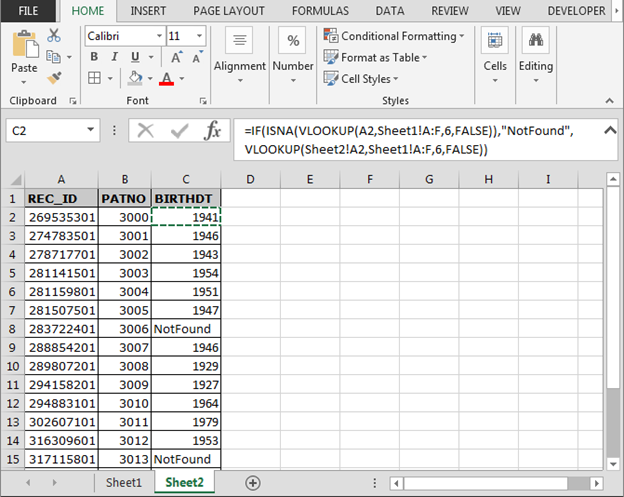# How to use IF, ISNA and VLOOKUP function in Excel?

In this article, we will learn how to use Excel ISNA function with VLOOKUP to ignore the #N/A error while calculating the formula in MS Excel 2016.

Excel VLOOKUP function is used to look up and fetch data from a specific column of a table. The "V" stands for "vertical". Lookup values must appear in the first column of the table, with lookup columns to the right.

## How to use the Vlookup function in excel?

VLOOKUP function in Excel is used to look up and retrieve data from a specific column of a table.

Syntax:

 =VLOOKUP(lookup_value, table_array, col_index_num, [range_lookup])

## How to use the ISNA function in excel?

ISNA function used for checking the #N/A error while calculation by using any function.

Syntax:

 =ISNA(Formula)

## How to use the if function in excel?

IF function works on logic_test and returns values based on the result of the test.

Syntax:

 =IF(Logic_test, [Value_if_True], [Value_if_False])

Let’s get this with an example.

Here we have two sheets, we have to get the BIRTHDT from SHEET 1 table to SHEET 2 Table using the functions.

Write the formula in C2 cell of SHEET 2.

 =IF(ISNA(VLOOKUP(A2,Sheet1!A:F,6,FALSE)),”NotFound”, VLOOKUP(Sheet2!A2,Sheet1!A:F,6,FALSE))

Explanation:
Vlookup function in excel looks for the 6th column of the SHEET 1 matching  REC_ID in SHEET 1 from SHEET 2.
ISNA function in excel looks for the #NA error and passes it on to IF function.
IF formula in excel checks if any #NA error occurs, it print “NotFound” instead of the #NA error.

Copy the formula in other cells, select the cells including the first cell where the formula is already applied, use shortcut key Ctrl + D.As you can see in the above image, the formula is working fine. And the user can work without getting irritated by #NA error.

Hope you understood IF, ISNA and VLOOKUP function in Excel 2016, 2013 and 2010. There are more articles on VLOOKUP Examples and HLOOKUP Examples here.   Please do check more links here and if you have any unresolved query, please state that in the comment box below. We will help you.

Two-way table lookups - can't get the function to work

If anybody can help with this I'm trying to get the correct price from the table from the data entered in AB2 and AB3. The function used is in cell AB7.

Click here:- How to use ISNA function along with INDEX and MATCH function?

IF Statement combining multiple 'IF TRUE' conditions

Assuming that I have a worksheet with columns A,B,C and D on it, now I want an IF statement in column E which follows this argument:
IF A1 is "0" or "N/A", then show N/A, but if A1 is any other number, then B*(C+D)
I hope that's easy to follow. I have tried so hard to get this to work but just can't.
As ever, any help would be hugely appreciated.

Related Articles:

ISERROR and VLOOKUP function

IFERROR and VLOOKUP function

VLOOKUP Multiple Values

Partial match with VLOOKUP function

1.Is there a way to get a vlookup to replace an NA from another vlookup? I have 2 sheets, one is hr and one is our data, hr has the updated info and ours have some lines that the hr file does not. when searching for the changes, I do want to pull in information so it will be easier to copy and paste but I need all the info in a combined sheet that works automatically. I have it so it pulls in the HR data but I need all the nas to fill in with our data if they appear. Please tell me this is possible!

•Yes, of course, you can do it. Just wrap the first Vlookup with the IFERROR function. on the error part, put another VLOOKUP formula.
Like this. IFERROR(VLOOKUP(lookupValue,table1,col,0),VLOOKUP(lookupValue,table2,col,0))